How to visualise millions of data points in a web browser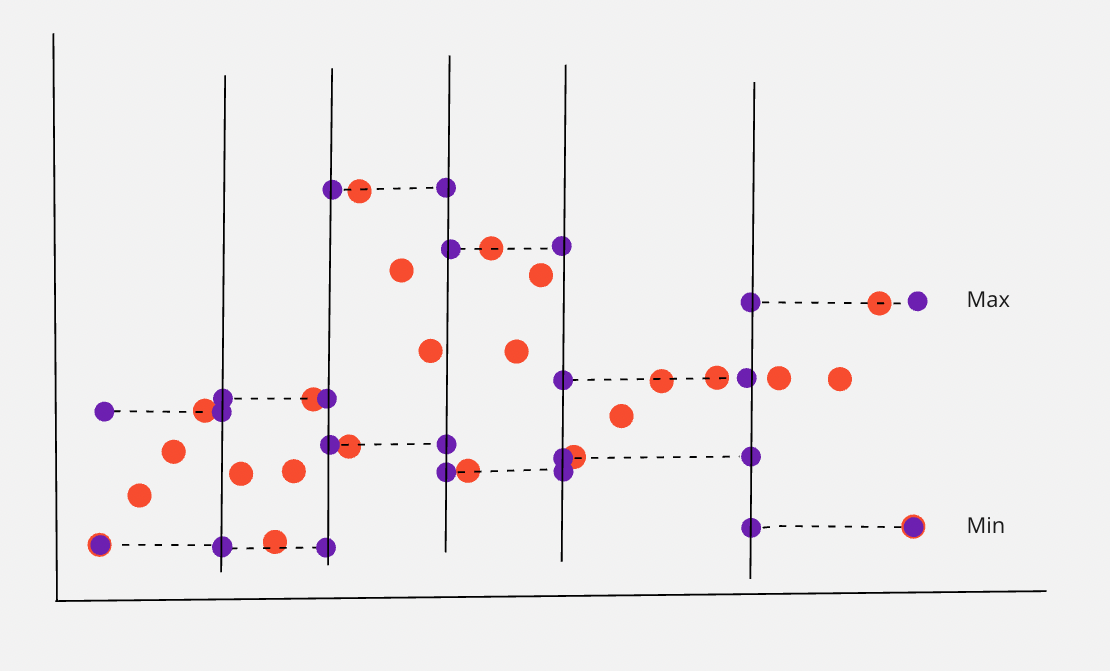In this blog post, we explain why Marple is so good and fast at drawing plots of large amounts of time series data.

How do we visualise so many data points? The short answer is: we don’t.

We smartly select which data points to show, and give a small twist to how we show them.

Like this, we manage to quickly load plots that are accurate enough to analyse your data.

### What’s the problem?

Data from sensors can quickly grow to large data sets.

People want to visualise this data but quickly realize that plotting millions of datapoints can be very slow.

It will cause your laptop or browser to freeze and you’ll have to select a small time range or be very careful what you want to plot.

### How can this problem be solved?

We solve this problem by cutting in the data that is actually visualised.

A fraction of the data points gives more or less the same plot. And by rendering fewer data points, the software becomes faster.

### How do we build this solution?

When you cut in the data that you use to draw a plot, you need to answer two questions.

1. How many data points do we show?
2. Which data points do we show?

How many data points do we show

This mostly depends on the preference of the user.

Loading 2000 data points per plot renders a plot with a resolution that is of sufficiently high quality. This is the standard resolution we at Marple use.

Lower or higher plot resolutions also do the job, as shown below.

Which data points do we show?

Setting a fixed number of data points we render in a plot, boils down to reducing the number of data points that are shown.

This means that we need to decide which data points we show.

It makes sense to divide the time range of the plot by the number of data points we show. In each resulting fraction (we call them ‘buckets’), we will need to select one data point that we show.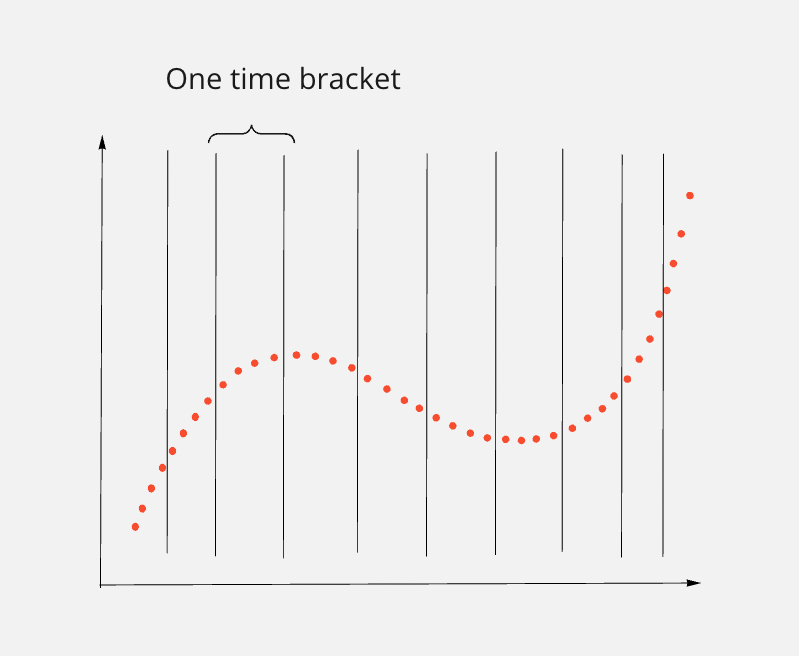A simplified example, in which we divide a plot of 40 data points into 10 buckets.

Do we show the first, last or average?

The noisier a measured signal gets, selecting either the first or the last data point in the bucket may give a skewed representation of the measurement.

Here is an example of a plot that, in full resolution, has 22 data points.

If we want to scale down the number of data points by a factor of 4, we can subdivide the datapoints into 6 time buckets.

If we select the first data point in each bucket, we end up with this plot.

First data point per bucket selected

Since we work with really low resolutions, we need to accept that the image of the plot will be skewed.

What is more troublesome, is that the trend we see is quite different compared to when we would select the last data point in each bucket.

There are other solutions, such as drawing a data point in the middle of each bucket that is the average or the mean of all data points in that bucket.

You could also try taking the average or mean of the first and last data point of the bucket, or some other function of the data points in the bucket.

Whatever you try, it is hard to ensure that the plot will be a sufficiently good representation of the measurement.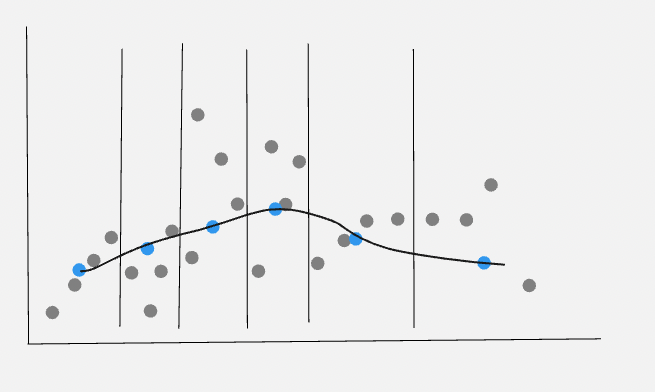A plot drawing a new data point that is the average of the first and last data point of the bucket

What if we don’t actually show data points?

The problem seems that, when selecting just one data point per bucket, you throw out the high frequency of your measurement.

The solution to this problem is obvious and non-obvious at the same time: make the entire bucket a data point!

Technically speaking, we no longer deal with points, but areas.

The upper bound of the bucket area is determined by the data point in the bucket with the highest value. The lower bound of the area is determined by the lowest value in the bucket.

Granted, in a very low resolution, this obviously looks a little ridiculous. But the further we increase the number of buckets a plot consists of, the better this solution works.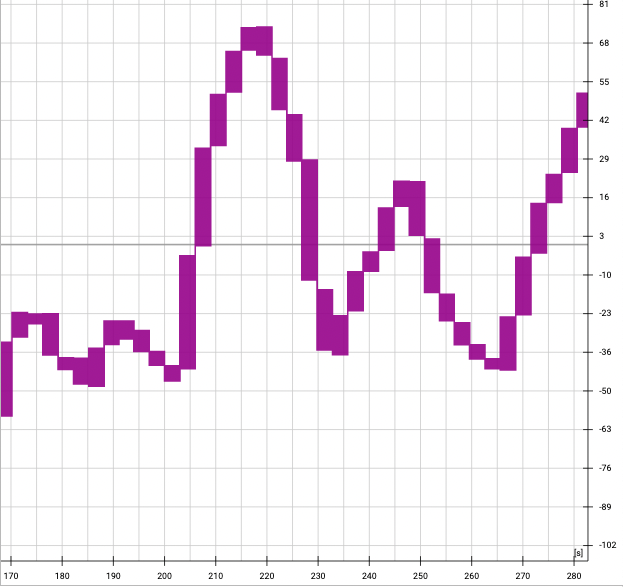When we set the plot resolution really low, you can clearly see the buckets in the plot. This is a screenshot of a plot resolution of 50 ‘data points’ per plot.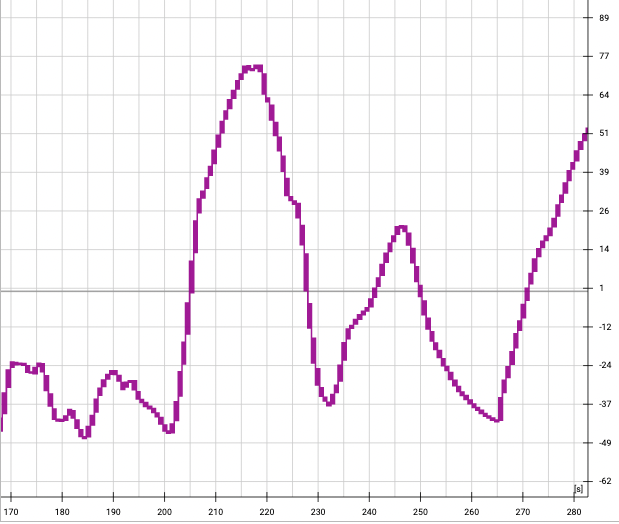Increasing the plot resolution (now at 200 ‘data points’ (but actually buckets) per plot), the plot starts looking more and more like a line

We’re still just rendering a fraction of the data points that were actually measured, but we already see a plot that gives us a good feeling of the measured signal.

By way of final touch, we can connect the buckets in a smoother, more gradual way. As a result, the plot looks no longer clunky.

When placing a cursor in the plot, we still show an actual data point that corresponds with where the cursor is.

And when you zoom in enough so that the data points in your zoom level drop below your chosen resolution, we of course show all data points.

### Conclusion

Plotting a lot of data points is hard to do in a performant way. That's why we don't do it.

Instead we do clever visual subsampling of the data in order to keep the plot performant.

In return you can really play with your data. This gives you the ability to intuitively explore your data and easily discover areas that are of further interest to you.

Just give Marple a try if you want to have a look at how this theory is put into practice! We’re always happy to hear what you think.